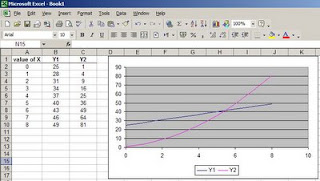### How to find where the two curves cross

Sachin, a colleague at work, came to me with this question: How can I find the value of the point where to lines cross in a graph?
Excel don't seem to have a build-in procedure to find such a value. The workaround I suggest is to use the Solver Add In.

Let's look at this example:The formulas to produce the graph are:
• in cells B2 to B10: =3*A2+25
• in cells C2 to C10: =A2^2+2*A2+1

Obviously Y1 crosses Y2 somewhere between X=4 and X=6. In order to find the exact value of X we'll use the Solver (in the menu bar: Tools-->Solver. If you can't see it, then you have to install the Add In).

Before opening the Solver copy the formulae in cell B10 and C10 to cells B12 and C12.In the "set target cells" window write (or select) B12.

In the "by changing cells"window write or select A12.

In the "subject to constraints" window add two constraints:

• B12 = C12 (the answer to our question)
• A12>=0 (in order to get a positive value)

Now, your sheet will look like this:Hit the "Solve" button, and you will get this: